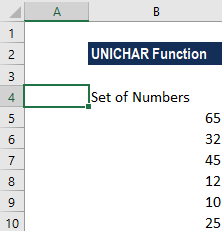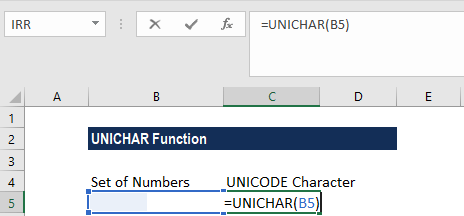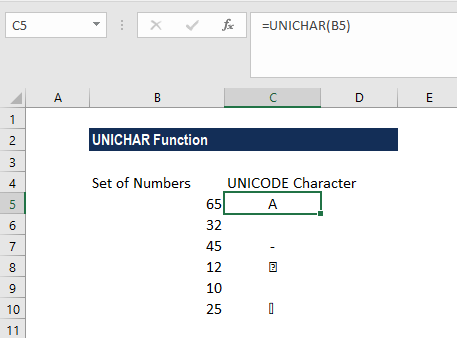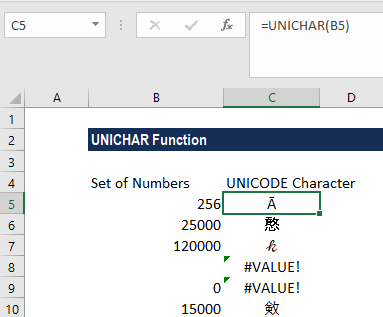# UNICHAR Function

Returns the Unicode character of a given number

## What is the UNICHAR Function?

The UNICHAR Function is categorized under Excel Text functions. UNICHAR gives the user a Unicode character of a specified number, where Unicode is a decimal number from 0 through 65535. The function was introduced in MS Excel 2013.

### Formula

=UNICHAR(number)

Where:

The number argument is an integer. It is a required input. It can be a number or a reference to a cell in a worksheet that contains the number. The UNICHAR function will convert the number given into a Unicode number that represents a character.

### How to use the UNICHAR Function in Excel?

To understand the uses of the UNICHAR function, let’s consider a few examples:

#### Example 1

Suppose we are given a set of data that we wish to convert to UNICODE characters. First, we will list down the data in a column, as shown below:In the adjacent column, we will enter the following formula:We get the results below:1. When the number given is 65, the UNICHAR function returns the character represented by the Unicode number 65 (uppercase A).
2. When the number given is 32, the UNICHAR function returns the character represented by the Unicode number 32 (space character).
3. If the number given is 45, the UNICHAR function returns the character represented by the Unicode number 45 (dash character).

#### Example 2

UNICHAR is an extension of the CHAR function. The CHAR function allows only numbers between 1 to 255. If we want to code numbers greater than 255, we need to use the UNICHAR function.We got a #VALUE! error is because we gave 0 in row 6 an unidentified number in row 5.

### The UNICHAR Function and Character Codes

Computers, as we are aware, work in numbers. Alphabets, special characters such as “&” and “#” are all stored and displayed by being assigned a unique number by the system.

Initially, the numbering system or the code page used was different. For example, there was the ANSI code system that was developed and used by Microsoft, whereas the Macintosh character set was developed and used by Macintosh computers.

Users were faced with problems when they tried converting the character codes from one system to another. To address the issue, a universal character set, which is known as the Unicode system, was developed. The system assigned a unique character code to all the characters used in all systems. It was designed to hold over a million code points. However, for compatibility, the first 255 code points of the Unicode system are the same as that of the ANSI system for Western language characters and numbers.

The UNICHAR function is useful as it helps to translate code page numbers into characters for files created on other types of systems using Unicode. UNICHAR can also be used with other Excel functions such as TRIM or SUBSTITUTE to remove unwanted characters from a worksheet.

### A few pointers about the UNICHAR Function

1. Based on the number, the Unicode character returned can be a string of characters, for example, in UTF-16 or UTF-8 codes. UTF refers to Unicode Transformation Format and includes UTF-1, UTF-7, UTF-8, UTF-16, and UTF-32.
2. The UNICHAR function will return an #N/A error when the Unicode numbers are partial surrogates and the data types are not valid.
3. #VALUE error occurs if the number is zero or the numbers fall outside the range allowed.
4. Remember, the uppercase and lowercase letters on the keyboard refer to different character codes, e.g., “A” = 65, “a”= 97.

Thanks for reading CFI’s guide to the Excel UNICHAR function. By taking the time to learn and master these functions, you’ll significantly speed up your financial analysis. To learn more, check out these additional CFI resources:

• Excel Functions for Finance